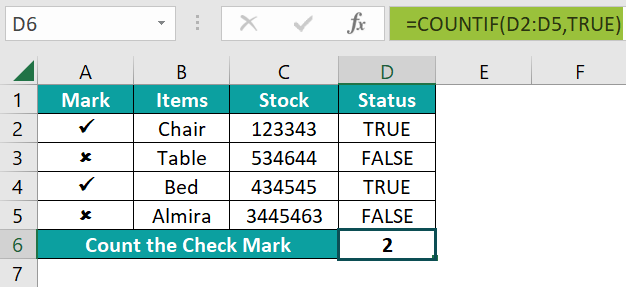# Check Mark in ExcelArticle byExcelMojo Team## What Is Check Mark / Tick (✓) Symbol In Excel?

The Check Mark / Tick (✓) Symbol in Excel is a special symbol that indicates whether the task is done or not. The Tick (✓) Symbol expresses the concept of “Yes.” The Check Mark in Excel is present in the Symbol option of the Insert tab.

For example, consider the table with the list of fruits. Now, let us learn how to add check mark in excel.

To add Check Mark in Excel, we should follow the below steps;

• Step 1: First, we should select the cell where we want to put the Check Mark. In this case, let us select cell B2.
• Step 2: Next, go to the Insert tab.
• Step 3: Then, click on the down arrow button of the Symbol option.
• Step 4: Now, select the Symbol option from the Symbols group.
• Step 5: The window called Symbol pops up.
• Step 6: Next, set the font as Wingdings from the Symbol group.
• Step 7: Then, select the Tick ( ✓ ) Symbol with the box.
• Step 8: Click the Insert button.
• Step 9: The result is shown in cell B2 as shown in the following image.

To insert the check mark in other cells, simply drag using the auto-fill excel option.

Likewise, we can use check mark in excel.

###### Key Takeaways
• Check Mark is a special symbol used to indicate whether the task is done or not.
• To detect the character code for any symbol, select that Symbol from the Symbol Window and then check the corresponding Character Code box shown at the right end of the Symbol Window.
• When we are using the Character Function, i.e., CHAR function, the selected cells should be in the “Wingdings” font for desired results.
• The Autocorrect method is used when we regularly use the same type of symbols.

### How To Make A Check Mark In Excel?

The following steps are used to insert Check Mark in Excel.

1. To begin with, select the cell where we want to insert check mark.

2. Then, select the Insert tab.

3. Next, click on the Symbols option.

4. Then, select the Symbol option.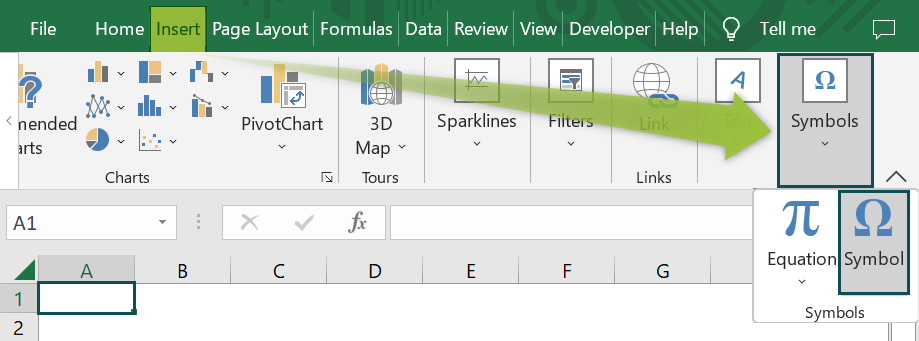5. Now, the window called Symbol pops up.

6. Set the font as Wingdings.

7. Next, select the Tick ( ✓ ) Symbol.

8. Then, click the Insert key.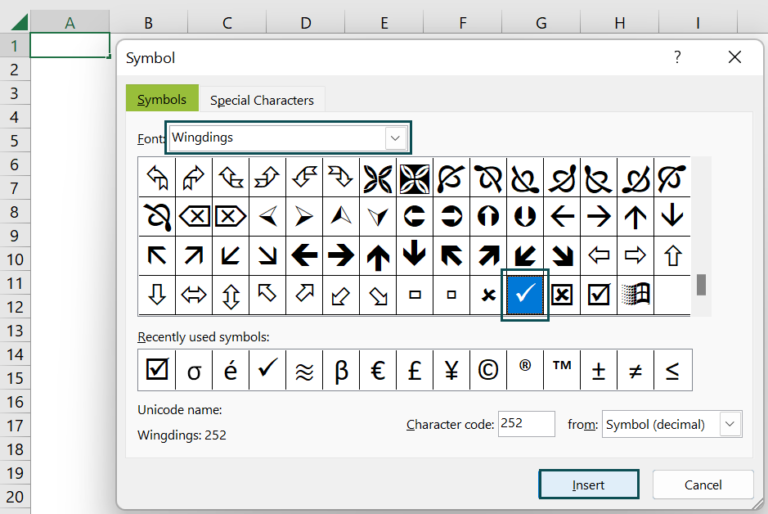9. The Tick ( ) Symbol will be inserted in the selected cell as shown in the below image.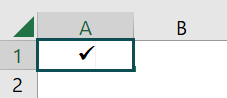Similarly, we can insert check mark in Excel.

### Top 7 Ways To Insert Check Mark (✓ Tick) In Excel

#### #1 – By Using The Tick ( ✓ ) Symbol Option In Excel

To Insert Check Mark by using Tick ( ✓ ) Symbol option in Excel, we should follow the below steps;

• Firstly, select the Insert tab, and then click on the down arrow button of the Symbol option.
• Then, select the Symbol option from the Symbols group.
• The pop-up window named Symbol opens up.
• Select the font as Wingdings from the Symbol group.
• Select the Tick ( ✓ ) Symbol, within the box or without the box.
• Click the Insert button.

Likewise, we can insert tick mark in excel.

#### #2 – Using The Character Code

To Insert Check Mark using the Character Code, we should follow the below steps;

• First, select the cell where we want to enter the Tick ( ✓ ) Symbol.
• Place the cursor in the cell where we would like to insert a Check Mark.
• Next, go to the Home tab and change the font settings to Wingdings.
• Hold the ALT key while typing the character code. In this case, the character code is 252.
• Then, press the Insert key.

Similarly, we can insert check mark in excel.

#### #3 – Use A Keyboard Shortcut Excel Key To Insert Tick Mark

The Keyboard Shortcut Excel Key to Insert Tick Mark are in two different ways;

• For Wingdings 2 font in Excel, the shortcut keys are:

Select the cells where we want to enter the Tick ( ✓ ) Symbol.

• For Webdings font in Excel, the shortcut keys are:

Select the cells where we want to enter the Tick ( ✓ ) Symbol.

Likewise, we can add check mark in excel.

#### #4 – Using CHAR Function

We can insert Check Mark by using the Character Code option in Excel with the following ways:

• To begin with, select the cell in which we want to enter the Tick ( ✓ ) Symbol. In this case, we need to select cells B1 & B2.
• Next, change the font to Wingdings.
• Then, enter the CHAR formula in Excel as shown in the following image. The full formula entered in cell B1 is =CHAR(0254), and in cell B2 is =CHAR(0252).

Similarly, we can insert check mark in excel.

#### #5 – Using The Option In Conditional Formatting

We can use the below steps to insert Check Mark using the option in Conditional Formatting;

• First, select the cells where we want to use Conditional Formatting option. In this case, the selected cells are in the range B2:B6.
• Go to the Home tab.
• Then, click on Conditional Formatting down arrow button in the Styles group.
• Select the Icon Sets Excel option from the drop-down list.
• Now, choose the 3 Symbols (Uncircled) option from the Indicators list from the drop-right list.
• The Symbols appear on the selected cells. The (×) symbol shows a salary of less than ‘\$30,000,’ the (!) symbol shows a salary greater than and equal to ‘\$30,000’ and less than ‘\$45,000,’ and the (✓) symbol shows a salary greater than and equal to ‘\$45,000.’
• The Symbols appear on the selected cells.

Likewise, we can add check mark in excel.

#### #6 – Using The ASCII Code

To Insert Check Mark using the ASCII Code in Excel, we should use the code of the Check Mark obtained by using the ASCII character.

The ASCII Character of the Check Mark is Ü, and the ASCII Code of the Check Mark is 252.

#### #7 – From The Bullet Library

To Insert Check Mark using the Bullet Library in Excel,

• Select the cells on which we want to use the Bullet Library in Excel.
• Go to the Home tab.
• Click the Bullet Library.
• Select the Tick ( ✓ ) Symbol.

### Important Things To Note

• The color of the Tick ( ✓ ) Symbol can be changed from the Home tab.
• As the Check Mark in Excel, there are other symbols where we can use the options manually whenever needed. That is called a checkbox.
• Similar to Radio buttons in excel, we can select ( ✓ ) Check Mark multiple times.
• Check Marks are commonly used to fill out surveys or application forms.

1. How to insert Check Mark in Excel?

To insert Check Mark in Excel, follow the following steps;

Step 1: Select the cell where we want to put the Check Mark. In this case, let us select cell A1.

Step 2: Go to the Insert tab.

Step 3: Click on the down arrow button of the Symbol option.

Step 4: Select the Symbol option from the Symbols group.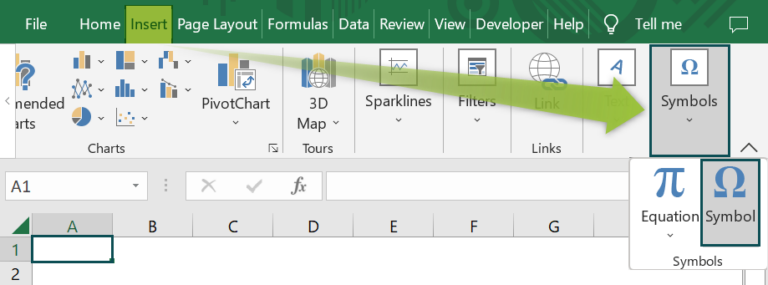Step 5: The window called Symbol pops up.

Step 6: Set the font as Wingdings from the Symbol group.

Step 7: Select the Tick ( ✓ ) Symbol, which is without the box.

Step 8: Click the Insert button.The result is shown in cell A1 as shown in the following image.2. What are the methods used to insert check mark?

There are 7 methods with which we can insert check mark in excel. They are;

• By Using The Tick ( ✓ ) Symbol Option In Excel
• Using the Character Code
• Use a Keyboard Shortcut excel key to Insert Tick Mark
• Using Char Functions
• Using the Option in Conditional Formatting
• Using the ASCII Code
• From the Bullet Library

3. How to count the number of Check Mark in an Excel Spreadsheet?

To count the Check Mark in Excel using the COUNTIF function, follow the following steps;

Step 1: First, select the cell where we want to put the Check Mark. In this case, the selected cell is cell A1.

Step 2: Next, go to the Insert tab.

Step 3: Click on the down arrow button of the Symbol option.

Step 4: Then, select the Symbol option from the Symbols group.

Step 5: The window called Symbol pops up.

Step 6: Now, set the font as Wingdings from the Symbol group.

Step 7: Select the Tick ( ✓ ) Symbol without the box.

Step 8: Then, click the Insert button.

Step 9: The result is shown in cell A1 in the following image.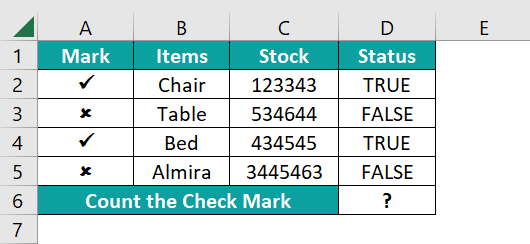Step 10: Next, set status in column D as TRUE or FALSE.

Step 11: Then, enter the COUNTIF formula in cell D6.

Step 12: The entered complete formula is =COUNTIF(D2:D5,TRUE) in cell D6.

Step 13: Press enter, and the result is shown in cell D6 as ‘2’, as shown in the below image.